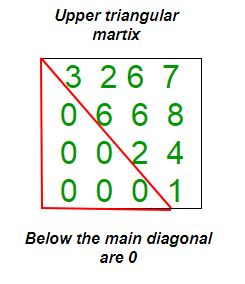GeeksforGeeks App
Open AppBrowser
Continue

# Python Program to check if matrix is upper triangular

Given a square matrix and the task is to check the matrix is in upper triangular form or not. A square matrix is called upper triangular if all the entries below the main diagonal are zero.Examples:

```Input : mat = {{1, 3, 5, 3},
{0, 4, 6, 2},
{0, 0, 2, 5},
{0, 0, 0, 6}};
Output : Matrix is in Upper Triangular form.

Input : mat = {{5, 6, 3, 6},
{0, 4, 6, 6},
{1, 0, 8, 5},
{0, 1, 0, 6}};
Output : Matrix is not in Upper Triangular form.```

## Python3

 `# Python3 Program to check upper``# triangular matrix.` `# Function to check matrix``# is in upper triangular``def` `isuppertriangular(M):``    ``for` `i ``in` `range``(``1``, ``len``(M)):``        ``for` `j ``in` `range``(``0``, i):``            ``if``(M[i][j] !``=` `0``):``                    ``return` `False``    ``return` `True``    ` `# Driver function.``M ``=` `[[``1``,``3``,``5``,``3``],``    ``[``0``,``4``,``6``,``2``],``    ``[``0``,``0``,``2``,``5``],``    ``[``0``,``0``,``0``,``6``]]` `if` `isuppertriangular(M):``    ``print` `(``"Yes"``)``else``:``    ``print` `(``"No"``)` `# This code is contributed by Anurag Rawat`

Output:

`Yes`

Time Complexity: O(n2), where n represents the number of rows and columns of the matrix.
Auxiliary Space: O(1), no extra space is required, so it is a constant.

Please refer complete article on Program to check if matrix is upper triangular for more details!

My Personal Notes arrow_drop_up